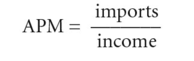# average propensity to import

## Average Propensity to Import

The amount of money a person spends on imports as a percentage of total income. For example, if one makes \$50,000 and spends \$10,000 on imported goods, the average propensity to import is 20%. It should not be confused with the marginal propensity to import.

## average propensity to import (APM)

the fraction of a given level of NATIONAL INCOME that is spent on IMPORTS:Alternatively, imports can be expressed as a proportion of DISPOSABLE INCOME. See also PROPENSITY TO IMPORT, MARGINAL PROPENSITY TO IMPORT.

Mentioned in ?
References in periodicals archive ?
The terms r, sd, mmr, apm, m, and rm denote international reserves, variability measure of the variations in the balance of payments, money market rate, the average propensity to import, the level of imports and workers' remittances.
The sign of the average propensity to import variable is not clear from the existing literature.
16] denote the long run parameters of the cointegration relationship of the variables reserves, variations in the balance of payments, money market rate, average propensity to import, the level of imports and remittances respectively and the value of [[beta].
The set of variables includes international reserves, measure of the variations in the balance of payments, the money market rate, imports, average propensity to import and remittances.

Site: Follow: Share:
Open / Close